Courses

# NCERT Solutions Chapter 11 - Constructions (II), Class 9, Maths Class 9 Notes | EduRev

Created by: Indu Gupta

## Class 9 : NCERT Solutions Chapter 11 - Constructions (II), Class 9, Maths Class 9 Notes | EduRev

The document NCERT Solutions Chapter 11 - Constructions (II), Class 9, Maths Class 9 Notes | EduRev is a part of Class 9 category.
All you need of Class 9 at this link: Class 9

Exercise 11.2

1. Construct a triangle ABC in which BC = 7cm, ∠B = 75° and AB + AC = 13 cm.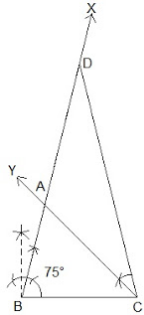Steps of Construction:
Step 1: A line segment BC of 7 cm is drawn.
Step 2: At point B, an angle ∠XBC is constructed such that it is equal to 75°.
Step 3: A line segment BD = 13 cm is cut on BX (which is equal to AB+AC).
Step 3: DC is joined and ∠DCY = ∠BDC is made.
Step 4: Let CY intersect BX at A.
Thus, ΔABC is the required triangle.

2. Construct a triangle ABC in which BC = 8cm, ∠B = 45° and AB - AC = 3.5 cm.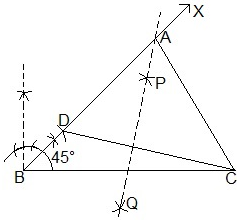Steps of Construction:
Step 1: A line segment BC = 8 cm is drawn and at point B, make an angle of 45° i.e. ∠XBC.
Step 2: Cut the line segment BD = 3.5 cm (equal to AB - AC) on ray BX.
Step 3: Join DC and draw the perpendicular bisector PQ of DC.
Step 4: Let it intersect BX at point A. Join AC.
Thus, ΔABC is the required triangle.

3. Construct a triangle PQR in which QR = 6cm, ∠Q = 60° and PR – PQ = 2cm.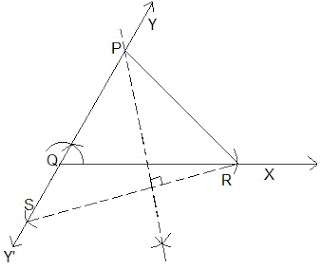Steps of Construction:
Step 1: A ray QX is drawn and cut off a line segment QR = 6 cm from it.
Step 2:. A ray QY is constructed making an angle of 60º with QR and YQ is produced to form a line YQY'
Step 3: Cut off  a line segment QS = 2cm  from QY'. RS is joined.
Step 5: Draw perpendicular bisector  of RS intersecting QY at a point  P. PR is joined.
Thus, ΔPQR is the required triangle.

4. Construct a triangle XYZ in which ∠Y = 30°, ∠Z = 90° and XY + YZ + ZX = 11 cm.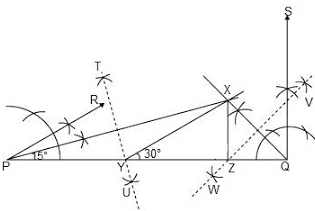Steps of Construction:
Step 1: A line segment PQ = 11 cm is drawn. (XY + YZ + ZX = 11 cm)
Step 2: An angle, ∠RPQ = 30° is constructed at point A and an angle ∠SQP = 90° at point B.
Step 3: ∠RPQ and ∠SQP are bisected . The bisectors of these angles intersect each other at point X.
Step 4: Perpendicular bisectors TU of PX and WV of QX are constructed.
Step V: Let TU intersect PQ at Y and WV intersect PQ at Z. XY and XZ are joined.
Thus, ΔXYZ is the required triangle.

5. Construct a right triangle whose base is 12cm and sum of its hypotenuse and other side is 18 cm.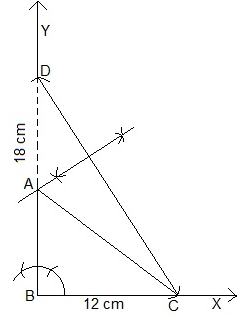Steps of Construction:

Step 1: A ray BX is drawn and a cut off a line segment BC = 12 cm is made on it.
Step 2: ∠XBY = 90° is constructed.
Step 3: Cut off a line segment BD = 18 cm is made on BY. CD is joined.
Step 4: Perpendicular bisector of CD is constructed intersecting BD at A. AC is joined.
Thus, ΔABC is the required triangle.

,

,

,

,

,

,

,

,

,

,

,

,

,

,

,

,

,

,

,

,

,

,

,

,

,

,

,

,

,

,

,

,

;